Scalabium SoftwareKnowledge for your independence'.HomeDelphi and C++Builder tips

#56: To play with colors

Sometimes in run-time you need play with colors. For example, you have the some color value and you want to make it more dark or light. The next two function were written for it.

function Dark(Col: TColor; Percent: Byte): TColor;
var R, G, B: Byte;
begin
R := GetRValue(Col);
G := GetGValue(Col);
B := GetBValue(Col);
R := Round(R*Percent/100);
G := Round(G*Percent/100);
B := Round(B*Percent/100);
Result := RGB(R, G, B);
end;

function Light(Col: TColor; Percent: Byte): TColor;
var R, G, B: Byte;
begin
R := GetRValue(Col);
G := GetGValue(Col);
B := GetBValue(Col);
R := Round(R*Percent/100) + Round(255 - Percent/100*255);
G := Round(G*Percent/100) + Round(255 - Percent/100*255);
B := Round(B*Percent/100) + Round(255 - Percent/100*255);
Result := RGB(R, G, B);
end;

Published: December 30, 1999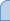See also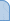MAPIMail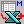DBExport toolsParadox to MS Access converter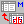DBISAM ViewerSMMsg suiteViewer for MS Outlook Messages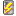SMImport suiteDBISAM Password RecoveryMail parser (ActiveX)Metafile Convert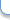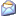Contact to webmasterCopyright© 1998-2022, Scalabium Software. All rights reserved. webmaster@scalabium.com